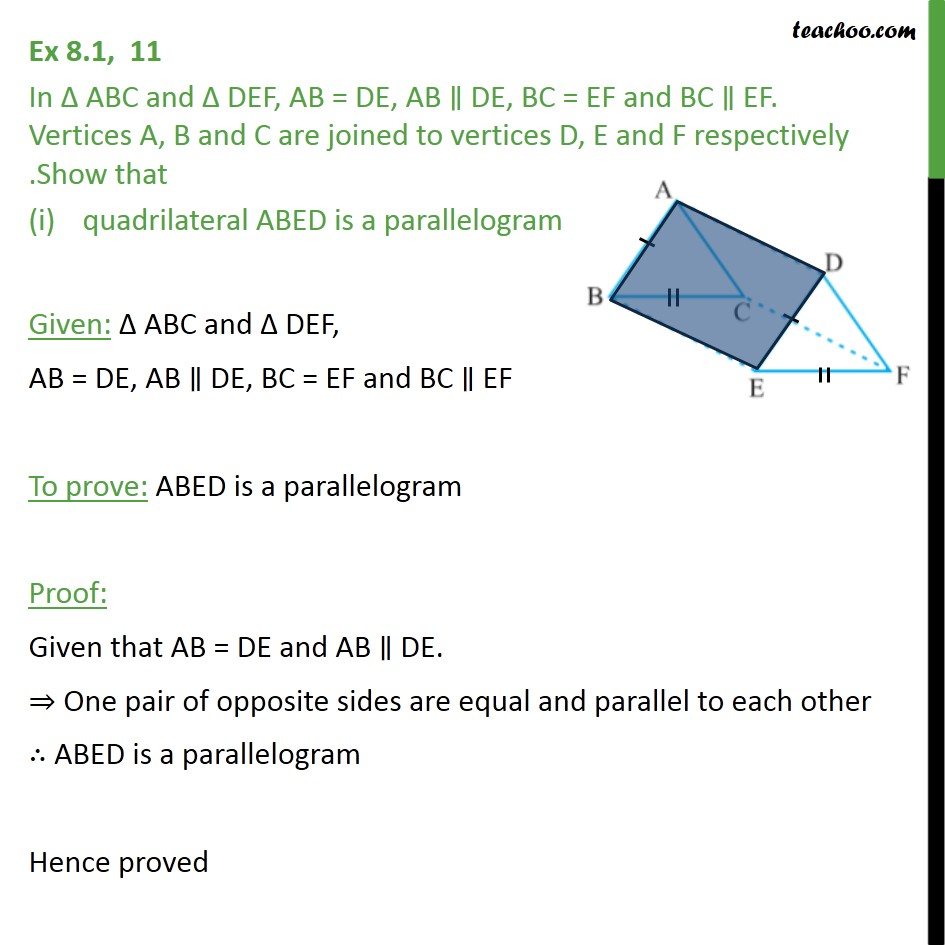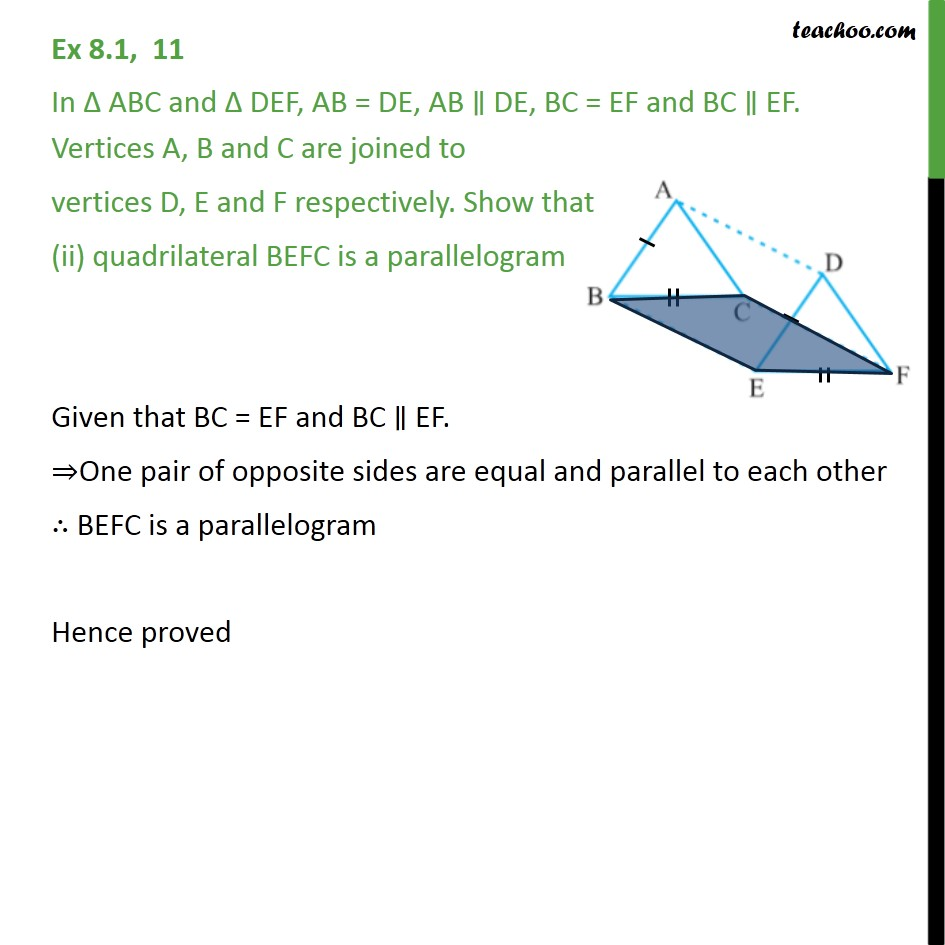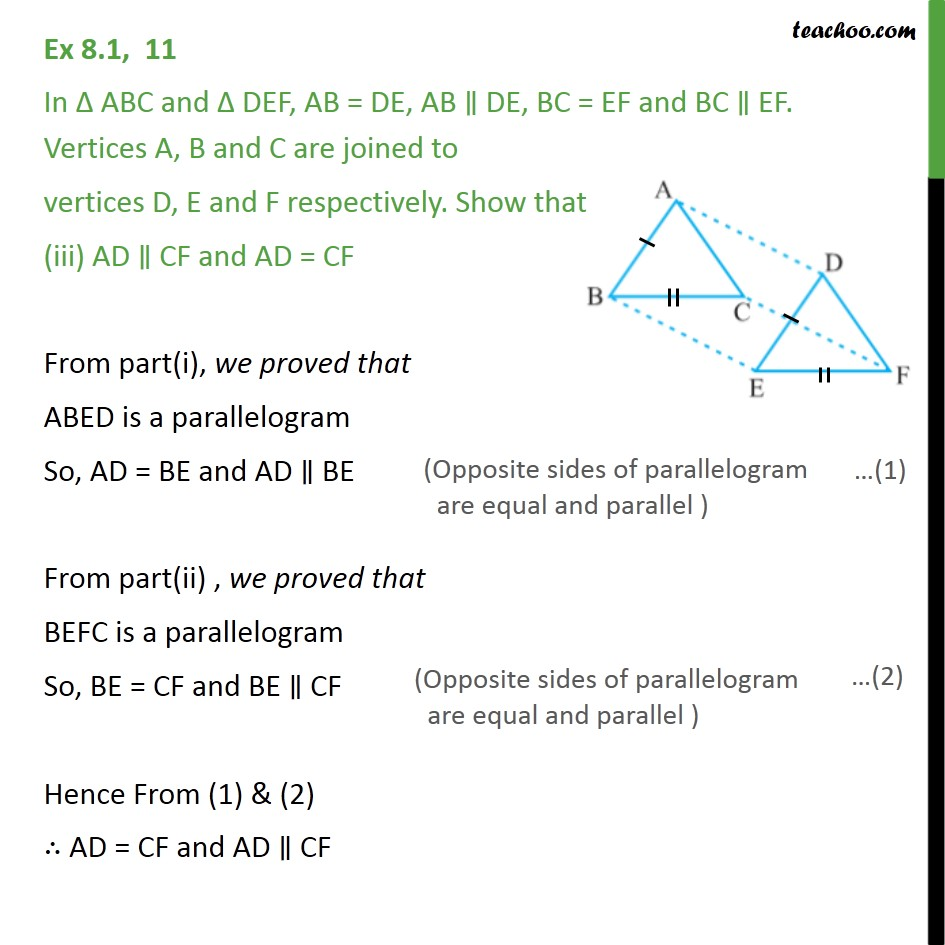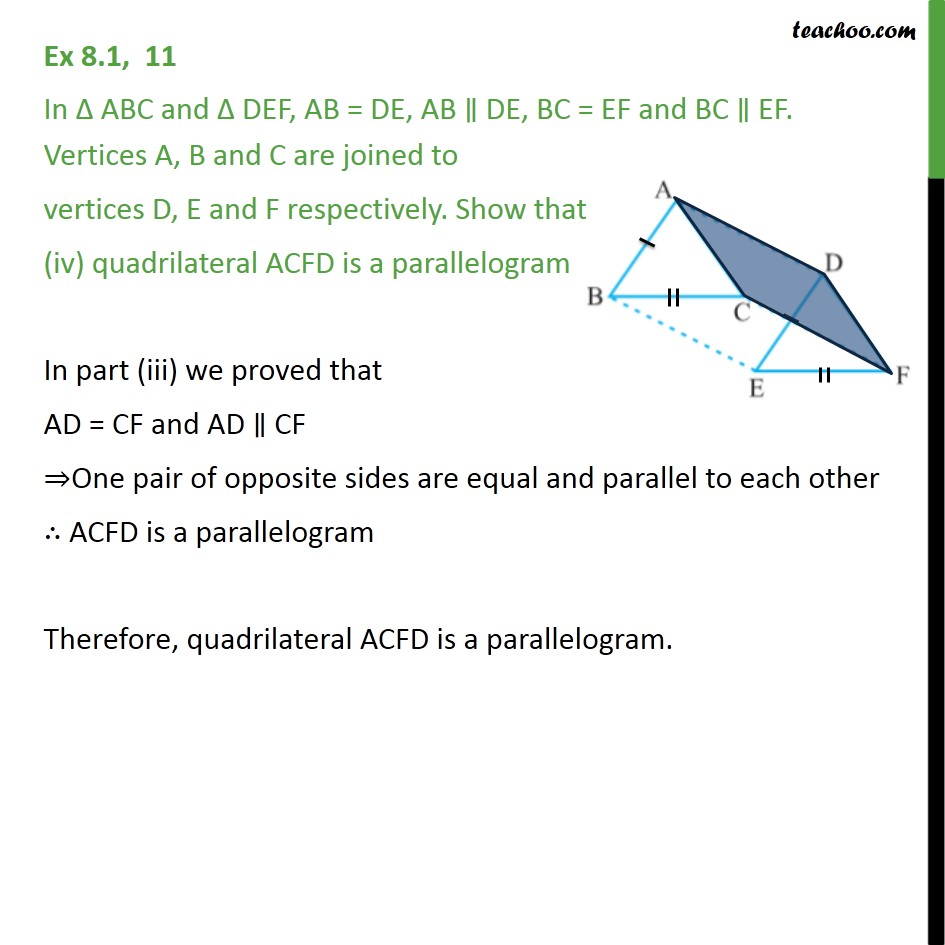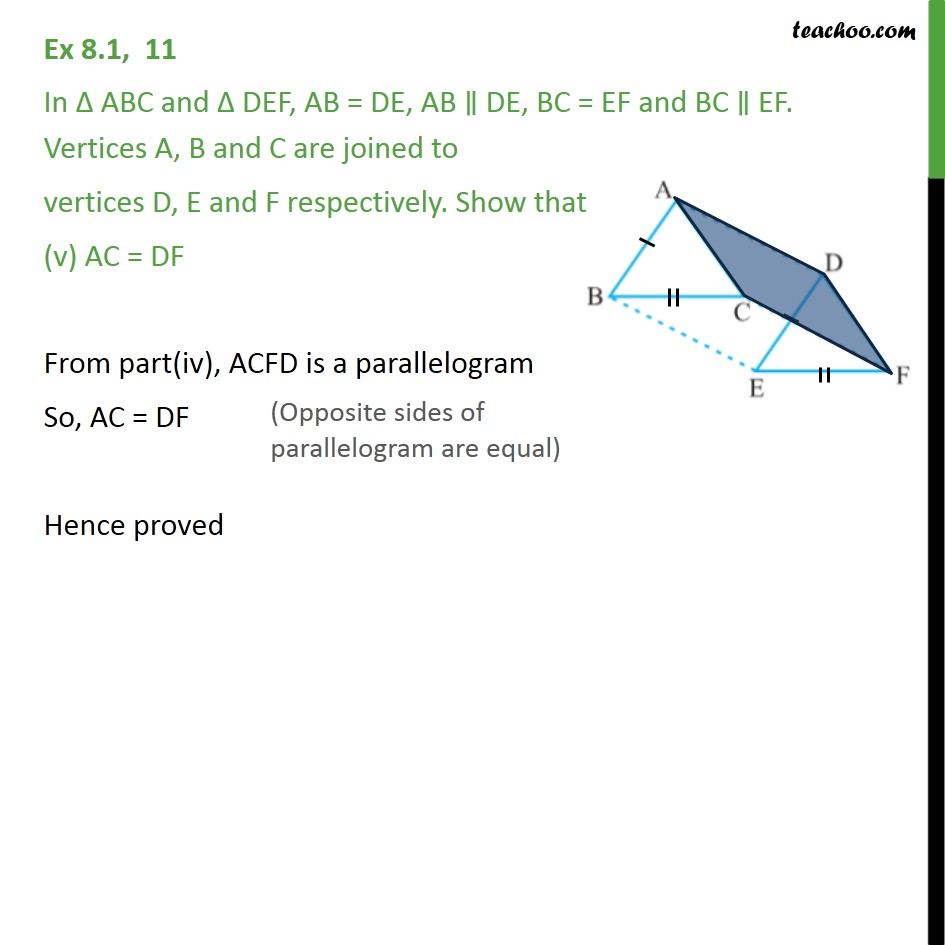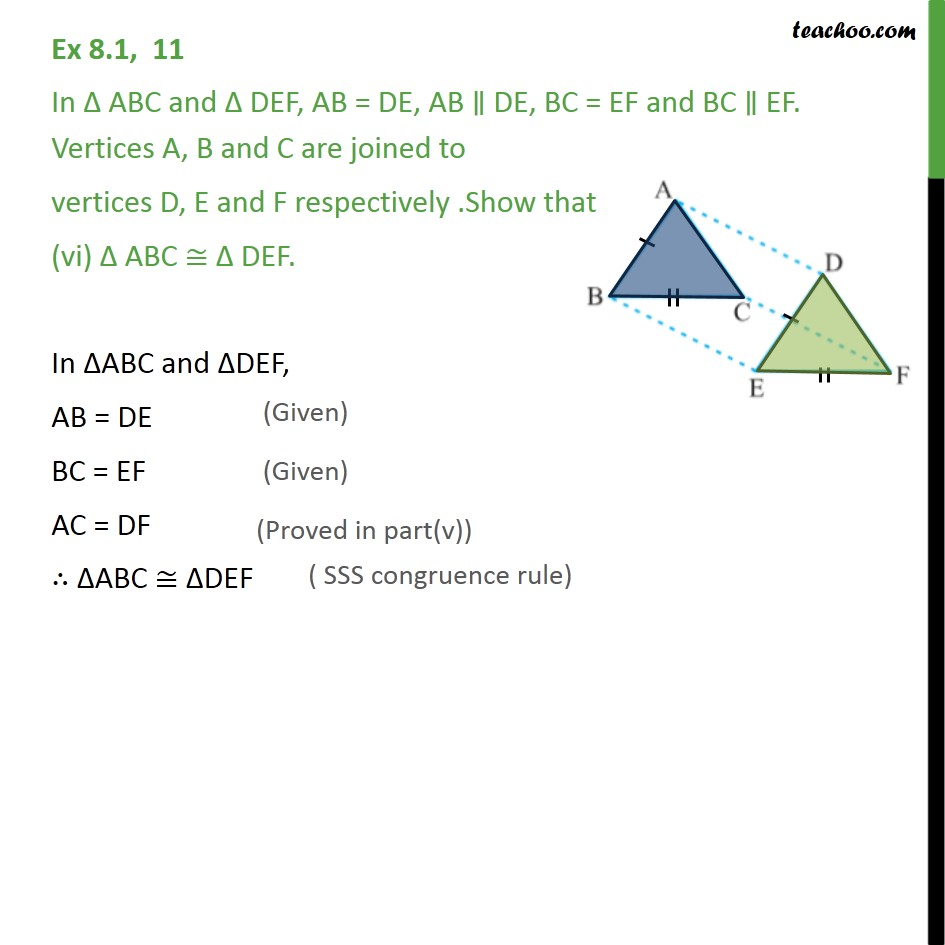1. Chapter 8 Class 9 Quadrilaterals
2. Serial order wise
3. Ex 8.1

Transcript

Ex 8.1, 11 In Δ ABC and Δ DEF, AB = DE, AB ∥ DE, BC = EF and BC ∥ EF. Vertices A, B and C are joined to vertices D, E and F respectively .Show that quadrilateral ABED is a parallelogram Given: Δ ABC and Δ DEF, AB = DE, AB ∥ DE, BC = EF and BC ∥ EF To prove: ABED is a parallelogram Proof: Given that AB = DE and AB ∥ DE. ⇒ One pair of opposite sides are equal and parallel to each other ∴ ABED is a parallelogram Hence proved Ex 8.1, 11 In Δ ABC and Δ DEF, AB = DE, AB ∥ DE, BC = EF and BC ∥ EF. Vertices A, B and C are joined to vertices D, E and F respectively. Show that (ii) quadrilateral BEFC is a parallelogram Given that BC = EF and BC ∥ EF. ⇒One pair of opposite sides are equal and parallel to each other ∴ BEFC is a parallelogram Hence proved Ex 8.1, 11 In Δ ABC and Δ DEF, AB = DE, AB ∥ DE, BC = EF and BC ∥ EF. Vertices A, B and C are joined to vertices D, E and F respectively. Show that (iii) AD ∥ CF and AD = CF From part(i), we proved that ABED is a parallelogram So, AD = BE and AD ∥ BE From part(ii) , we proved that BEFC is a parallelogram So, BE = CF and BE ∥ CF Hence From (1) & (2) ∴ AD = CF and AD ∥ CF Ex 8.1, 11 In Δ ABC and Δ DEF, AB = DE, AB ∥ DE, BC = EF and BC ∥ EF. Vertices A, B and C are joined to vertices D, E and F respectively. Show that (iv) quadrilateral ACFD is a parallelogram In part (iii) we proved that AD = CF and AD ∥ CF ⇒One pair of opposite sides are equal and parallel to each other ∴ ACFD is a parallelogram Therefore, quadrilateral ACFD is a parallelogram. Ex 8.1, 11 In Δ ABC and Δ DEF, AB = DE, AB ∥ DE, BC = EF and BC ∥ EF. Vertices A, B and C are joined to vertices D, E and F respectively. Show that (v) AC = DF From part(iv), ACFD is a parallelogram So, AC = DF Hence proved Ex 8.1, 11 In Δ ABC and Δ DEF, AB = DE, AB ∥ DE, BC = EF and BC ∥ EF. Vertices A, B and C are joined to vertices D, E and F respectively .Show that (vi) Δ ABC ≅ Δ DEF. In ΔABC and ΔDEF, AB = DE BC = EF AC = DF ∴ ΔABC ≅ ΔDEF

Ex 8.1

About the AuthorDavneet Singh
Davneet Singh is a graduate from Indian Institute of Technology, Kanpur. He has been teaching from the past 10 years. He provides courses for Maths and Science at Teachoo.